Home

ARTICLE 61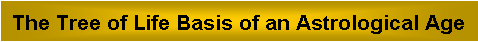by

Stephen M. Phillips

Flat 4, Oakwood House, 117-119 West Hill Road. Bournemouth. Dorset BH2 5PH. England.

Website: http://smphillips.mysite.com

Abstract

 Sacred geometries embody the number 216 because it is the gematria number value of Geburah, one of the 10 Sephiroth of the Kabbalistic Tree of Life. For example, 216 points & lines surround the centre of the 3-dimensional Sri Yantra, whilst 216 yods intrinsic to the seven enfolded, regular polygons of the inner Tree of Life line their 47 sectors when the latter are tetractyses. This means that the 70 polygons enfolded in every 10 overlapping Trees of Life have 2160 such boundary yods. This, too, is a parameter of holistic systems, e.g., 2160 lines & triangles surround the axes of the five Platonic solids when their vertices and centres of faces are joined to their centres. This number is known in astronomy as the number of years it takes for the axis of the Earth to rotate through 30° as the Sun moves through each of the 12 astrological signs into which the celestial sphere is divided (a so-called ‘astrological era’). The Earth’s axis rotates 360° in the 12-stage cycle of a Platonic Year of 12×2160 = 25,920 years. A rotation of 1° requires 72 years. The number 72 is the number value of Chesed, the Sephirah opposite Geburah in the Tree of Life. As a geometrical symbol for the 12 signs of the Zodiac, spaced 30° apart, the dodecagon is the seventh and last regular polygon in the inner Tree of Life. The number of yods surrounding the centres of two joined, Type A dodecagons is 140, which is the gematria number value of Masloth, the Mundane Chakra of Chokmah. The number value 73 of Chokmah is the number of yods in a Type A dodecagon. What is remarkable is that the Sphere of the Zodiac is the astrological identification of Masloth! If, like a year and the zodiac, an astrological era is 12-fold, then the 2160 yods that surround the centres of the Type B dodecagons enfolded in 12 overlapping Trees symbolise the 2160 years of an era represented by these 12 Trees. The Chinese calendar can accommodate both a 10-fold and a 12-fold cycle because it groups years within a 10-year cycle (10 Celestial Stems) and a 12-year cycle (12 Earthly Branches). Curiously, the number (1680) of oscillations of each whorl of the UPA remote-viewed by Charles Leadbeater over a century ago is the same as the number of years during which the Earth’s axis rotates through an angle equal within a discrepancy of 0.12% to its current angle of inclination of about 23½°.

1

Table 1. Gematria number values of the ten Sephiroth in the four Worlds.

 SEPHIRAH GODNAME ARCHANGEL ORDER OF ANGELS MUNDANE CHAKRA 1 Kether (Crown)   620 EHYEH (I am)   21 Metatron (Angel of the Presence)   314 Chaioth ha Qadesh (Holy Living Creatures)   833 Rashith ha Gilgalim First Swirlings. (Primum Mobile)   636 2 Chokmah (Wisdom)   73 YAHWEH, YAH (The Lord)   26, 15 Raziel (Herald of the Deity)   248 Auphanim (Wheels)   187 Masloth (The Sphere of the Zodiac)   140 3 Binah (Understanding)   67 ELOHIM (God in multiplicity)   50 Tzaphkiel (Contemplation of God)   311 Aralim (Thrones)   282 Shabathai Rest. (Saturn)   317 Daath (Knowledge)   474 4 Chesed (Mercy)   72 EL (God)   31 Tzadkiel (Benevolence of God)   62 Chasmalim (Shining Ones)   428 Tzadekh Righteousness. (Jupiter)   194 5 Geburah (Severity)   216 ELOHA (The Almighty)   36 Samael (Severity of God)   131 Seraphim (Fiery Serpents)   630 Madim Vehement Strength. (Mars)   95 6 Tiphareth (Beauty)   1081 YAHWEH ELOHIM (God the Creator)   76 Michael (Like unto God)   101 Malachim (Kings)   140 Shemesh The Solar Light. (Sun)   640 7 Netzach (Victory)   148 YAHWEH SABAOTH (Lord of Hosts)   129 Haniel (Grace of God)   97 Tarshishim or Elohim   1260 Nogah Glittering Splendour. (Venus)   64 8 Hod (Glory)   15 ELOHIM SABAOTH (God of Hosts)   153 Raphael (Divine Physician)   311 Beni Elohim (Sons of God)   112 Kokab The Stellar Light. (Mercury)   48 9 Yesod (Foundation)   80 SHADDAI EL CHAI (Almighty Living God)   49, 363 Gabriel (Strong Man of God)   246 Cherubim (The Strong)   272 Levanah The Lunar Flame. (Moon)   87 10 Malkuth (Kingdom)   496 ADONAI MELEKH (The Lord and King)   65, 155 Sandalphon (Manifest Messiah)   280 Ashim (Souls of Fire)   351 Cholem Yesodeth The Breaker of the Foundations. The Elements. (Earth)   168

 The Sephiroth exist in the four Worlds of Atziluth, Beriah, Yetzirah and Assiyah. Corresponding to them are the Godnames, Archangels, Orders of Angels and Mundane Chakras (their physical manifestation). This table gives their number values obtained by the ancient practice of gematria, wherein a number is assigned to each letter of the alphabet, thereby giving a number value to a word that is the sum of the numbers of its letters. Numbers from the table are written in boldface in the article.

2

1. The number 216 of Geburah in sacred geometries

The inner form of the Tree of Life consists of two sets of seven polygons:

triangle, square, pentagon, hexagon, octagon, decagon & dodecagon.

When their 47 sectors are tetractyses, the seven enfolded polygons contain 264 yods (Fig. 1). Of the 264 yods in the seven enfolded polygons, 47 hexagonal yods are at the centres of tetractyses. This leaves 217 yods (41 corners & 176 hexagonal yods) lining their sides (Fig. 2). One of these is the topmost corner of the hexagon. It coincides with the lowest corner of the hexagon that is part of the inner form of the next higher, overlapping Tree of Life. 216 yods that are intrinsic to each Tree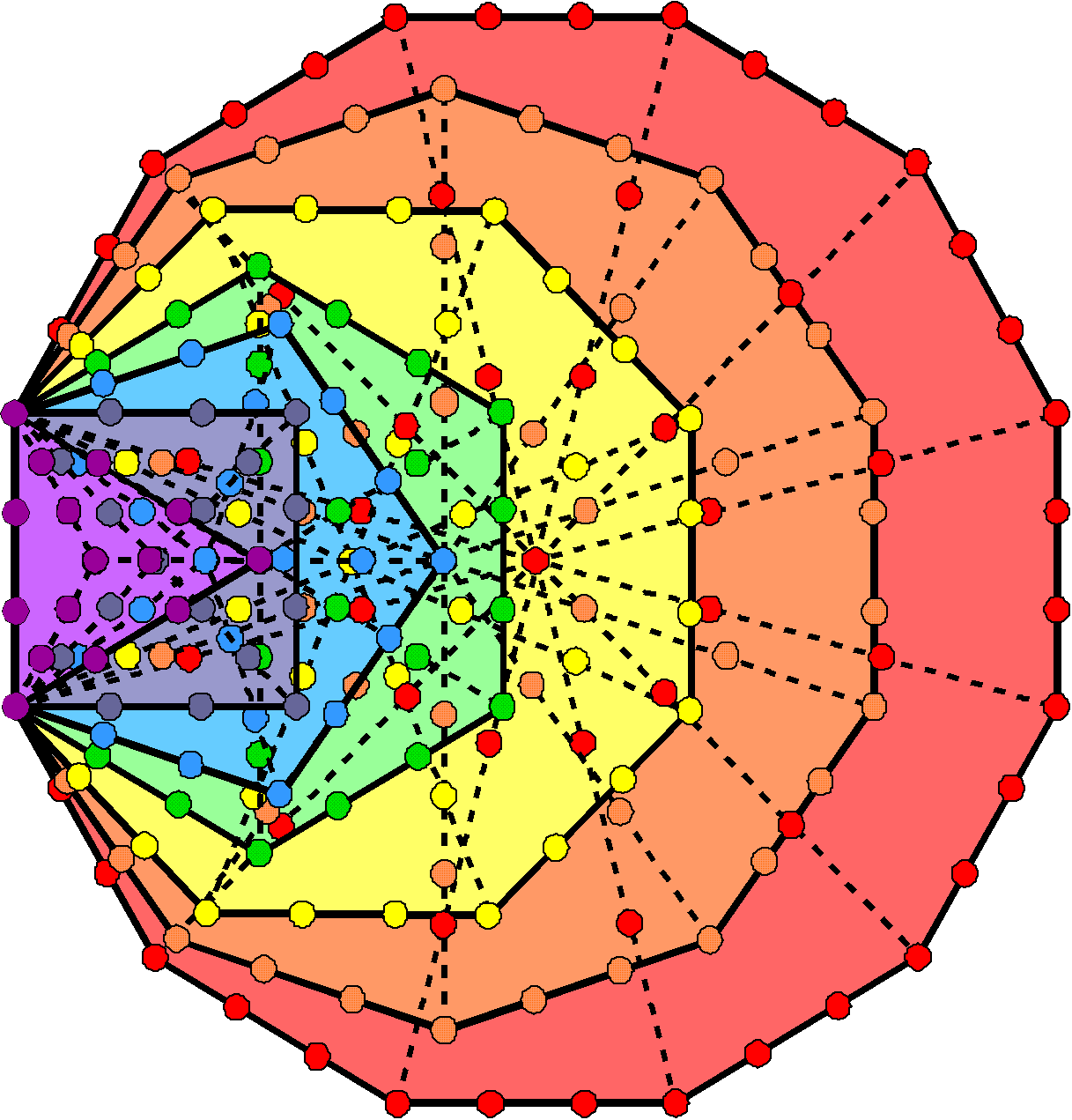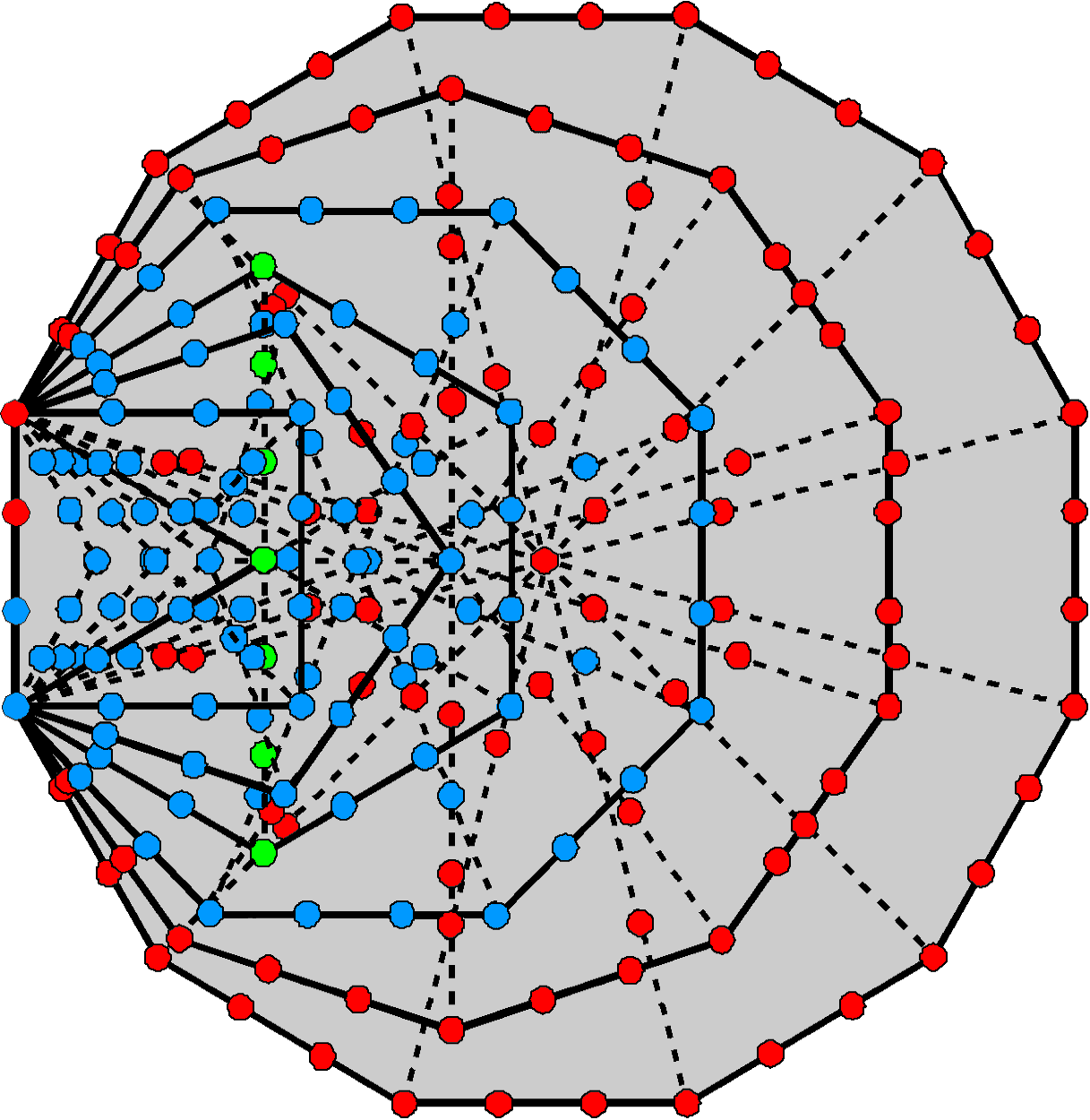Figure 1. The seven enfolded polygons of the inner Tree of Life contain 264 yods. Figure 2. 217 yods line the 47 tetractyses in the 7 enfolded polygons. Figure 3. 105 intrinsic, boundary yods are associated with the first 5 polygons (blue) and with the last 2 polygons (red).

shape the tetractyses making up each half of the inner form of successive Trees of Life.  216 is the number value of Geburah, the sixth Sephirah of Construction, counting from the base of the Tree of Life. The seven enfolded polygons have 36 corners, where 36 is the number value of ELOHA, the Godname of Geburah. This is an example of the conjunction of the numbers of the same Sephirah in the same sacred-geometrical context.

The number 217 measures another property of the inner Tree of Life. The seven enfolded polygons have 47 tetractys sectors containing 264 yods, of which 41 are corners and 223 are hexagonal yods. Outside the root edge are (223–2=221) hexagonal yods, of which four yods lie on the vertical axis of the hexagon and are shared with the outer Tree of Life (see next paragraph). This leaves (221–4=217) hexagonal yods outside the root edge which are intrinsic to the seven enfolded polygons. The number 217 is the intrinsic hexagonal yod population of the inner Tree of Life external to the root edge.

When the 16 triangles in the outer Tree of Life are tetractyses, seven yods lie on the Pillar of Mercy. They coincide with the seven green yods on the vertical axis of the hexagon. These are the only yods that the outer Tree shares with its inner form in which each yod is the same type in both cases. The 217 yods on the sides of the 47 tetractyses consist of these seven shared yods and 210 (=21×10) yods that are intrinsic to the inner Tree. It shows how EHYEH, the Godname of Kether with number value 21, prescribes those yods unshared with the outer Tree that shape the tetractyses making up its inner form.

Tabulated below in Table 2 are the numbers of intrinsic yods outside the root edge on the sides of the tetractyses in each polygon:

Table 2. Number of intrinsic boundary yods outside root edge in the 7 separate Type A polygons.

 Root edge Triangle Square Pentagon Hexagon Octagon Decagon Dodecagon 2 + 2 = 4 11 17 22 16 37 46 57 Total = 103 103

103 intrinsic yods (coloured blue) line the 25 tetractyses in the first five enfolded polygons and 103 intrinsic yods (coloured red) line the 22 tetractyses in the last two enfolded polygons. 103 is the number value of SABAOTH ("Hosts"), part of the Godnames of Hod and Netzach (see Table 1). Two blue yods in the root edge can be associated with the former set and two red yods can be associated with the latter. This means that the 210 intrinsic yods divide into two equal sets of 105 yods (Fig. 3). It is easily verified that these two sets are unique — there are no other combinations of polygons with 103 yods.

Let us next compare this property of the inner Tree of Life with the Sri Yantra. The 3-dimensional Sri Yantra consists of four layers of 42 triangles (21 triangles in each half) that surround the central triangle (coloured green) above them. Its three corners represent the three-fold Godhead, or trimurti (Hindi:

3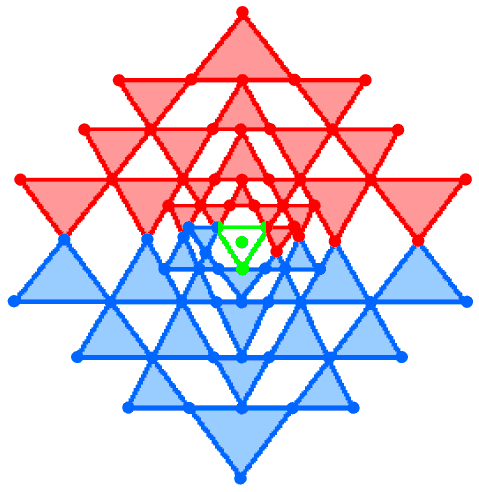Figure 4. The 21 triangles in each half of the 3-d Sri Yantra surrounding the seven points & lines in the central triangle and bindu comprise 105 points & lines.

त्रिमू्र्ति, translation: "Trinity"), of Shiva, Brahma & Vishnu. A dot, or bindu, representing the Absolute, the source of all manifestation, both physical and superphysical. The central triangle comprises three corners & three sides. The 42 triangles comprise 84 corners (42 in each half) and 126 sides (63 in each half). Each half of the 3‑dimensional Sri Yantra is shaped by (42+63=105) corners & sides (Fig. 4). Together with the seven green points & lines making up the bindu and the central triangle, (105+105+7=217) points & lines constitute the Sri Yantra. 216 points & lines surround the central point, 210 (=21×10) belonging to the 42 triangles.

We find that the same number (217) that measures the shape of the sectors of the seven enfolded polygons quantifies those geometrical elements that shape the Sri Yantra. Moreover, the seven yods in the inner Tree of Life shared with its outer form correspond to the seven points & lines making up the bindu and central triangle. It is remarkable that the set of seven enfolded polygons divide uniquely into two subsets of complete polygons with 105 yods that correspond to the 105 points & lines in the 21 triangles in each half of the Sri Yantra. Both sacred geometries also display the division: 217 = 1 + 216.

These correspondences demonstrate the equivalence of the Sri Yantra and the inner Tree of Life. Their geometrical dissimilarity hides the fact that they embody the same numbers quantifying analogous properties. These numbers are defining parameters of all holistic systems/sacred geometries.

2. How the number 217 determines the dimension of E8

As well as shaping the inner Tree of Life and the 3-dimensional Sri Yantra, the number 217 has both an arithmetic and a geometric significance vis-à-vis the dimension 248 of E8, the rank 8, exceptional Lie group that plays a pivotal role in one of the two heterotic superstring theories.

Arithmetic
The dimension of SO(32) and E8×E8, the symmetry groups describing the anomaly-free interactions between the two types of heterotic superstrings, is 496. As the third perfect number (1), this number is the sum of its nine factors: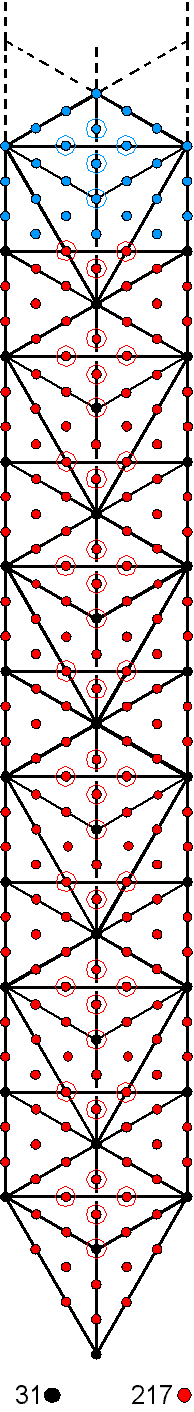496 = 1 + 2 + 4 + 8 + 16 + 31 + 62 + 124 + 248.

Therefore, 248 is the sum of the eight smallest factors of 496:

 248 = (1+2+4+8+16) + (31+62+124) = 31 + 217,

where 217 = 31 + 62 + 124 = 7×31. As 31 = 20 + 21 + 22 + 23 + 24, 62 = 2×31 and 124 = 22×31, 217 may be written as the following sum of powers of 2:

217 = 20 + 23 + 24 + 26 + 27,

so that 216 = 23 + 24 + 26 + 27.

Geometric
Constructed from 67 triangles, the 5-tree contains 280 yods when they are tetractyses, where 67 is the number of Binah and 280 is the number value of Sandalphon, the Archangel of Malkuth (Fig. 5). There are 248 yods up to Chesed of the fifth Tree of Life (see also here), where 248 is the number of Raziel, the Archangel of Chokmah. This SL is the 31st SL, where 31 is the number value of EL, the Godname of Chesed. Therefore, the 248 yods comprise 31 SLs (shown in Fig. 5 as black yods) and 217 hexagonal yods (shown as red yods). Remarkably, as the number of corners of tetractyses containing 248 yods, the number 31 determines the number 217 both geometrically, when the tetractyses are combined, and arithmetically, because 217 = 31×7, i.e., 31 separate tetractyses have 217 hexagonal yods.

Figure 5

3. The zodiacal age measured by sacred geometry

As Figure 2 shows, 217 yods line the sides of the 47 tetractyses making up each half of the inner Tree of Life. As the topmost corner of the hexagon in the inner Tree of Life coincides with the lowest corner of the hexagon in the inner form of the next higher, overlapping Tree of Life, 216 boundary yod are intrinsic to the set of seven polygons enfolded in successive Trees. 216 is the number value of

4

Geburah. The number of yods on the boundaries of the 47n tetractyses in the 7n polygons enfolded in the n-tree ≡ B(n) = 216n + 1, where "1" denotes the shared corner of the hexagon. The 470 tetractyses in the 70 polygons enfolded in the 10-tree are shaped by B(10) = 2160 yods that are intrinsic to them. In fact, the 70 polygons enfolded in every 10 Trees are composed of 470 tetractyses lined by 2160 yods. What is the significance of the emanation of 10 Trees? According to the discussion of the Cosmic Tree of Life (CTOL) in many previous articles, 91 Trees of Life map the seven cosmic planes of consciousness. These Trees should be regarded as emanating in 10 stages, each a cycle of 10 Trees, the last Tree emanated in each stage being, as its source, the first Tree of the next stage. The number 2160 represents a measure of each cycle, being the number of yods needed to shape the inner form of the 10 Trees emanated in each stage. Astronomers have calculated that the axis of the Earth rotates in a circle once every 25,920 years approximately (the so-called 'Platonic Year'). In this precession of the equinox, the axis rotates through 30° about every 2160 years, creating an astrological age that is dominated by one of the 12 zodiacal signs (2). It makes the stars move west to east about one degree about every 72 years. These numbers are only approximate because the lengths of the ages are decreasing with time as the rate of precession is increasing. Therefore, no two ages are of equal length. With this qualification, the astronomical number 2160 may be seen as a terrestrial counterpart of the parameter 2160 associated with the 10‑fold emanation of CTOL. It may be regarded as an example of the hermetic principle "As above, so below." Remarkably, the first four enfolded polygons have 72 intrinsic yods on the sides of their 17 tetractyses, the last three polygons having 144 such yods lining their 22 tetractyses, so that the 216 boundary yods intrinsic to each set of seven polygons naturally divide into a set of 72 and a set of 144 yods. The number value of Chesed is 72 and the number value of Geburah, the next Sephirah, is 216, where 216 = 23 + 24 + 26 + 27. Notice that this can be re-arranged as (23+26) + (24+27) = (8+64) + (16+128) = 72 + 144, which are the numbers of intrinsic yods lining the tetractyses in, respectively, the first four and the last three enfolded polygons of the inner Tree of Life. When geometry is sacred, it always exhibits a beautiful harmony with the properties of integers. The division 216 = 72 + 144 is found also in the 144 Polyhedron, which is part of the Polyhedral Tree of Life (see here). Formed from the disdyakis dodecahedron with 26 vertices, 72 edges & 48 faces by attaching tetrahedra to its faces, it has (26+48=74) vertices, (72 + 3×48 = 216) edges and (3×48=144) triangular faces. Its 216 edges comprise the 72 edges of the disdyakis dodecahedron and the extra 144 edges of the added tetrahedra. The 144 Polyhedron has 432 geometrical elements surrounding an axis passing through two opposite vertices. In terms of years, this number, which plays a part in Hindu chronology concerning the four yugas, corresponds to the period of 1/5 of an astrological age, or a 6° (1/60th circle) rotation of the Earth's axis. Notice that 144 (=122) is the 12th Fibonacci number after 0 and the only non-trivial, square, Fibonacci number.

Embodiment of the number of Tiphareth

According to Table 1, 103 yods outside the root edge line the 22 tetractyses in the decagon & dodecagon. This number does not include its centre, which the table counted as a corner of the pentagon. Let us, instead, count it with the decagon. Including the four yods in the root edge, there are 108 boundary yods in the last two polygons and 109 boundary yods in the first five polygons. The latter includes the corner of the hexagon shared with the hexagon belonging to the polygons enfolded in the next higher Tree. The number of boundary yods outside the root edge in the 5n polygons of the first five types enfolded in the n‑tree ≡ b(n) = 108n + 1. There are (b(10)=1081) such yods shaping the 50 polygons of these types enfolded on either side of the central pillar of the 10-tree. This is the gematria number of Tiphareth, the Sephirah at the centre of the Tree of Life! Although remarkable, the appearance of this number in the inner form of the 10-tree is not unexpected in view of the holistic character of this set of Trees, as demonstrated in many previous articles available on this website by the way in which the 10-tree is prescribed by Godnames and embodies superstring structural and dynamical parameters. Using the formula given above for B(n), there are also (B(5)=1081) yods lining the 235 tetractyses in the 35 polygons of all seven types enfolded in the 5-tree. This is discussed here. Therefore, the number of the Sephirah that divides the Tree of Life into two sets of five Sephiroth also divides 10 Trees into two sets of five Trees because 1081 boundary yods belong to the inner form of the lowest five Trees and 1081 yods (one yod being shared with the former set) belong to the inner form of the next five Trees.

The number of boundary yods outside the root edge in the first (5+5) polygons enfolded in the n-tree = 2b(n) = 2×(108n+1) = 216n + 2, where "2" denotes the top corners of the two hexagons enfolded in the nth Tree that coincide with the lowest corners of the hexagons enfolded in the (n+1)th Tree. The first (5+5) enfolded polygons have 50 sectors, showing how they are prescribed by ELOHIM, the Godname of Binah with number value 50. The (50+50) polygons of the first five types enfolded in the 10-tree have (50×10=500) tetractyses with 2160 boundary yods outside their root edges that are intrinsic to them. Compare this with the fact that the 70 polygons enfolded on either side of the 10-tree contain 2160 intrinsic boundary yods. It demonstrates the geometrical connection between the number values of Chesed, Geburah and Tiphareth — successive Sephiroth in the Tree of Life. It also shows how the same holistic parameters re-appear in different holistic subsets of a holistic system, defining their properties.

5

It is proved in #20 at Wonders of Superstrings that, when the vertices and face centres of the five Platonic solids are joined to their centres and their faces and all internal triangles divided into their sectors, there are 2160 sides & triangles that surround their axes passing through two opposite vertices and their centres. This means that, on average, 216 lines and triangles in each half of a Platonic solid surround its axis. Moreover, on average, 72 corners surround their axes. The presence in the regular polyhedra of the same parameters as were found above in the inner form of the 10-tree is evidence of their analogous, holistic character.

4. Tree of Life basis of an astrological age

We have just seen that the number 2160 is a measure of a holistic system, being a property of the 70 polygons enfolded in the 10-tree. The Platonic Year is divided into 12 eras, or astrological ages, each of length 2160 years. This number measures a 12-stage cycle of a complete rotation of the Earth's axis. Is it a property of the inner form, or part thereof, of 12 overlapping Trees of Life? If coincidence could be discounted as a plausible reason for its appearance, this would be an indication that the precession of the equinox, although having a well-understood astronomical cause, conforms to the universal pattern embodied in sacred geometry. Chance can be refuted by providing sufficient examples of the number 2016 or 1081 appearing in 12 Trees of Life to make this factor highly improbable. Figure 6 illustrates three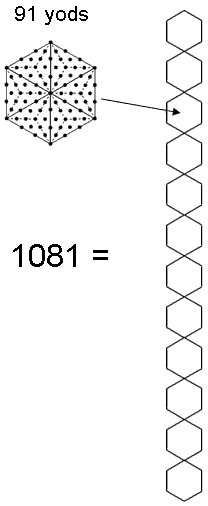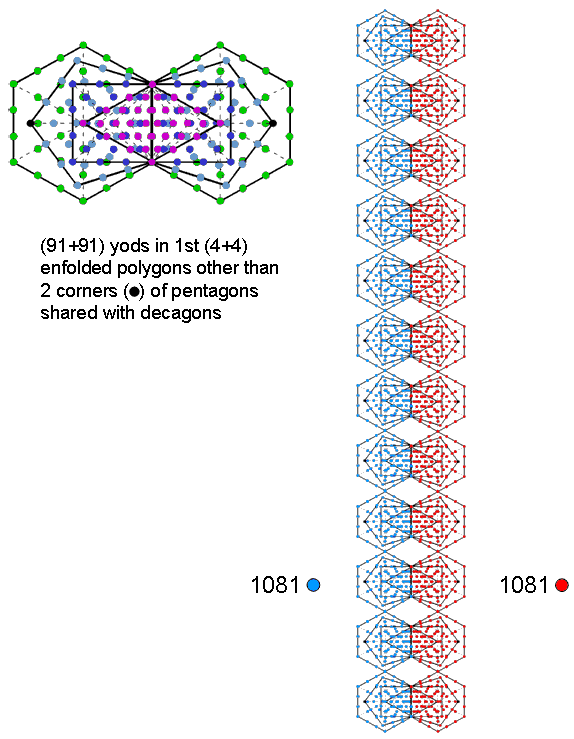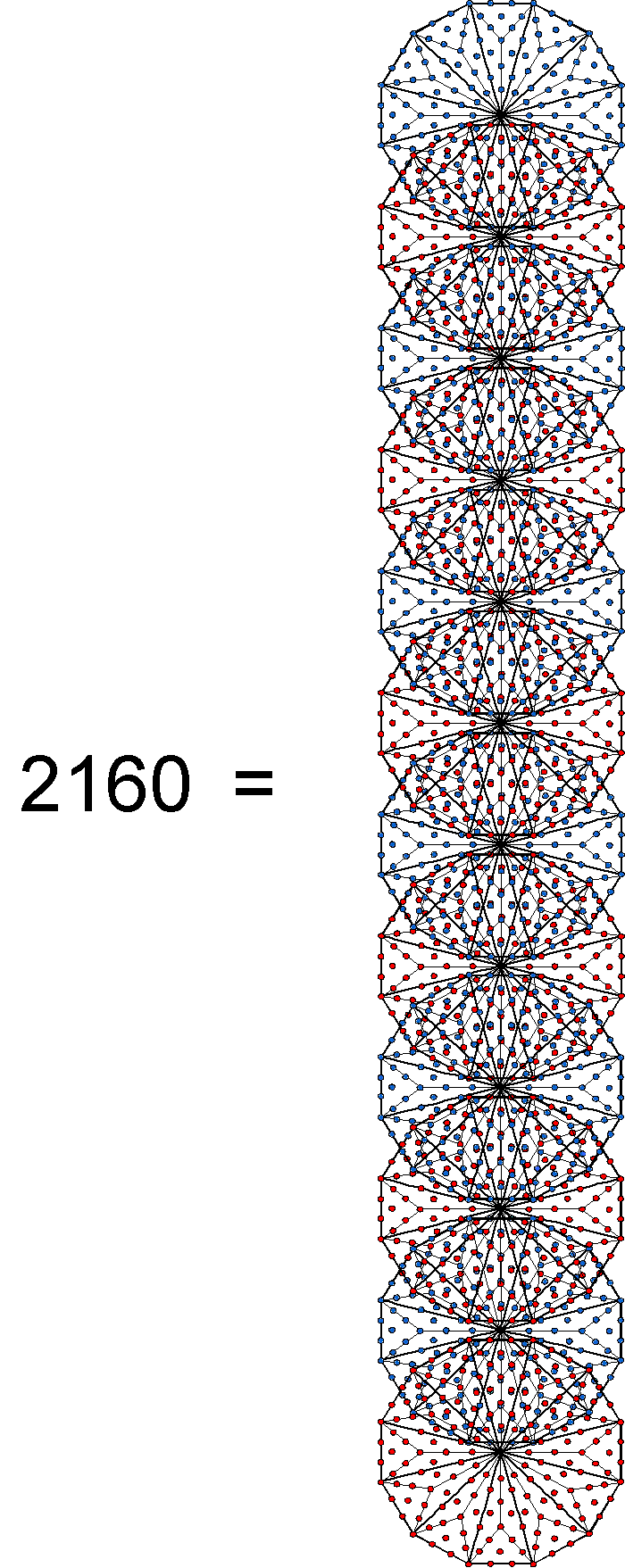A Type B hexagon has 91 yods. As its topmost corner is shared with the hexagon enfolded in the next higher Tree, 90 yods are intrinsic to it, The n hexagons enfolded in the n-tree contain (90n+1) yods. The 12 hexagons enfolded in the 12-tree have 1081 yods. This is the gematria number of Tiphareth (תפארת). The first four enfolded Type A polygons have 90 yods outside the root edge. The right-hand corner of the pentagon (black dot) coincides with the centre of the decagon. 89 yods outside the root edge of these polygons belong solely to them, although one yod (topmost corner of the hexagon) is shared with the hexagon enfolded in the next higher Tree. Associated with each set of the first four enfolded polygons are 91 such yods, one being shared. Associated with the 48 polygons of  the first four types enfolded on either side of the central axis of the 12-tree are (12×90 + 1= 1081). The topmost corner of each hexagon enfolded in the 12th Tree is shared with the hexagons enfolded in the 13th tree. 1080 yods are intrinsic to the 48 polygons in each set of 84 polygons enfolded in the 12-tree and do not belong to any of the remaining 36 polygons. (1080+1080=2160) intrinsic, unshared yods make up the first (4+4) polygons making up the inner form of 12 Trees. The Type B dodecagon has 181 yods. Surrounding its centre are 180 yods. Surrounding the centres of the dodecagons enfolded in 12 Trees of Life are (12×180=2160) yods.

Figure 6

6

of them. It shows that the number of years in an astrological age is embodied in 12 overlapping Trees of Life as the 2160 yods both intrinsic to the first four types of polygons enfolded in their inner form and unshared with any of the remaining types of polygons. It is also embodied in the last type of polygon making up the inner Tree of Life as the 2160 yods that surround the centres of the Type B dodecagons enfolded in the 12-tree. The sceptic's argument that this lacks significance because it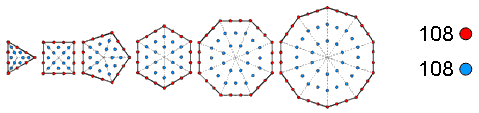Figure 7. As the number of Geburah, the 216 yods surrounding the centres of the first 6 separate polygons consist of 108 yods on their sides and 108 yods in their interiors.

is based upon cherry-picking ignores the fact that the geometrical context supposedly picked merely to generate the number 2160 also produces in various, natural ways the gematria number value 1081 of Tiphareth, the central Sephirah of the Tree of Life. What is the likelihood of that happening by chance? It is far too small to make the sceptic's vacuous dismissal plausible. Is it cherry picking to point out, as was done on the previous page, the simple, mathematical fact that 2160 lines and triangles surround the axes of the five Platonic solids when their vertices and face-centres are joined to their centres? Hardly. What is being encountered here is a spectacular example of how the gematria numbers of the Sephiroth are parameters of holistic systems. Is it coincidence that the first six separate polygons of the inner Tree of Life, which is shown in many sections of this website to constitute a holistic subset of the set of seven polygons, have 216 yods surrounding their centres made up of 108 yods on their sides and 108 yods in their interiors (Fig. 7)? This means that there is the same 1080:1080 division in yods in 10 such subsets as there is in the 12 pairs of the first four polygons shown in Figure 6? Is it yet another coincidence that this subset has 36 corners, where 36 is the number value of ELOHA, the Godname of Geburah, whose number is 216? No. Instead, what we have here is one of the patterns that characterises a holistic system re-appearing in part of it because this part, too, is holistic, exhibiting all the numbers of the Sephiroth in the four Kabbalistic Worlds.

The embodiment of the number 2160 by subsets of polygons belonging to the inner form of 12 Trees of Life suggests the representation by 12 Trees of Life of each 30 degree rotation of the earth's axis from one astrological age to the next, the Platonic Year spanning a complete 360° rotation of the Earth's axis being mapped by (12×12=144) Trees. The 12-fold division of the year is not, as astronomers might think, the product of early man noticing 12 full moons within one year — at least not for the Chinese. Their zodiac, Sheng Xiao, was based on a 12-year cycle, each year being named after an animal (2013 is the year of the Snake, ending January 14, 2014). Chinese astronomers divided the celestial sphere into 12 divisions in order to follow the orbit of Jupiter, whose period is 11.6 years. They were unaware of the Earth's precessional motion, the Greek astronomer-astrologer Hipparchus being regarded by most academics as the first to notice it around 127 B.C.E., although some scholars believe, controversially, that he more likely re-discovered it from his studies in Egypt. The Chinese divided a year into 12 periods and each day into 12 two-hour periods, or shi (the 12 "Earthly Branches," 地支). The 12-fold division in the calendar seems to have been the product of astronomical observation. For the Chinese, however, the phenomenon of precession did not influence it as they did not populate the celestial sphere with constellations.

Here is hard, mathematical evidence for a sacred-geometrical connection between the numbers 12 and 2160. The number 12 manifests elsewhere as the 12 roots of G2, the smallest exceptional Lie group, as the 12 notes between the tonic and octave found in the seven musical scales (see here) and as the 12 meridians of acupuncture (see Article 32). Perhaps, it is also revealed by the hidden, 12-fold division in the layout of Plato's books, which was recently discovered by Dr Jay Kennedy (see news item #4 here). In view of such occurrences, it should not seem so strange that the 12-sided dodecagon and 12 dodecagons enfolded in 12 overlapping Trees should embody a number that measures the time for the Earth's axis to rotate through 1/12th of a circle! What, however, is the Kabbalistic significance of 12 Trees, with each one mapping a 2½° rotation of the Earth's axis that, theoretically speaking, takes 180 years? Malkuth of the 12th Tree is Kether of the 10th Tree, the 65th SL, which is prescribed by ADONAI, the Godname of Malkuth, because its number value is 65. ADONAI can be said in this way to determine 12 Trees as well as 10 Trees. Constructed from tetractyses, 12 Trees contain 620 yods. 620 is the number value of Kether. The apex of the 12th Tree is the 76th SL, where 76 is the number value of YAHWEH ELOHIM, the Godname of Tiphareth. It is the 26th SL on the Pillar of Equilibrium, below which are 50 SLs on the two side pillars. 26 is the number value of YAHWEH and 50 is the number value of ELOHIM. The 12th Tree is the 80th from the top of CTOL (for the meaning of CTOL, see Maps of reality), where 80 is the number of Yesod. These are a few examples of how Kabbalistic numbers determine 12 Trees. The

7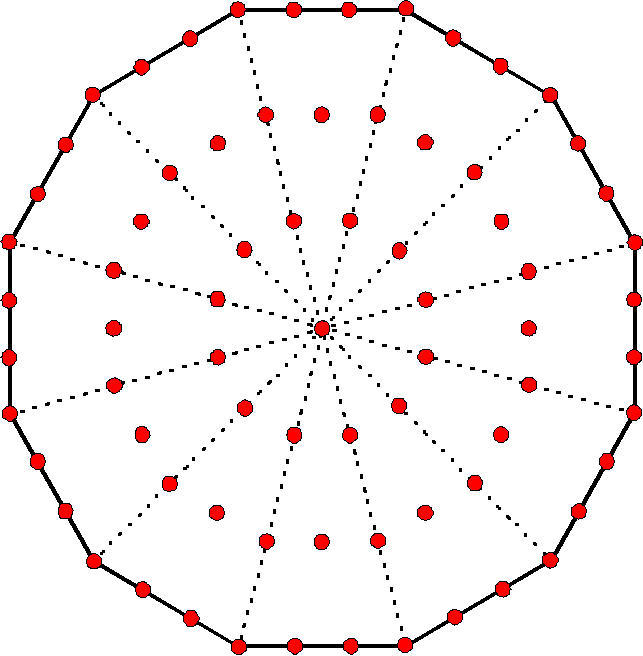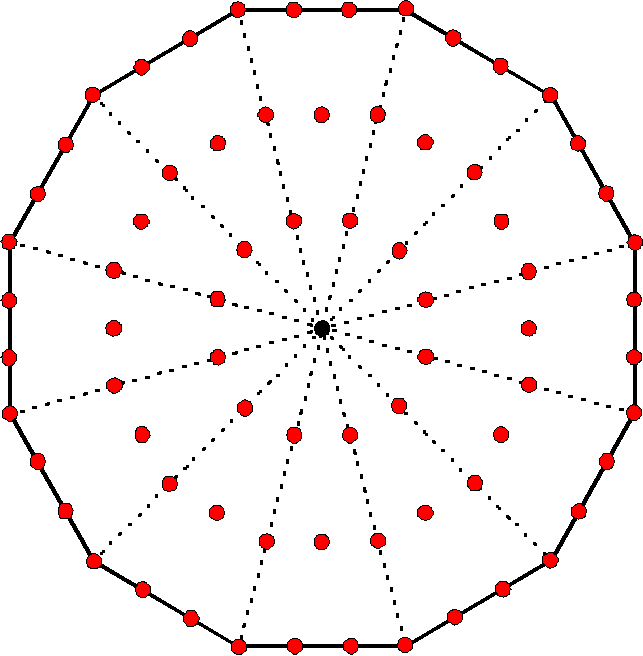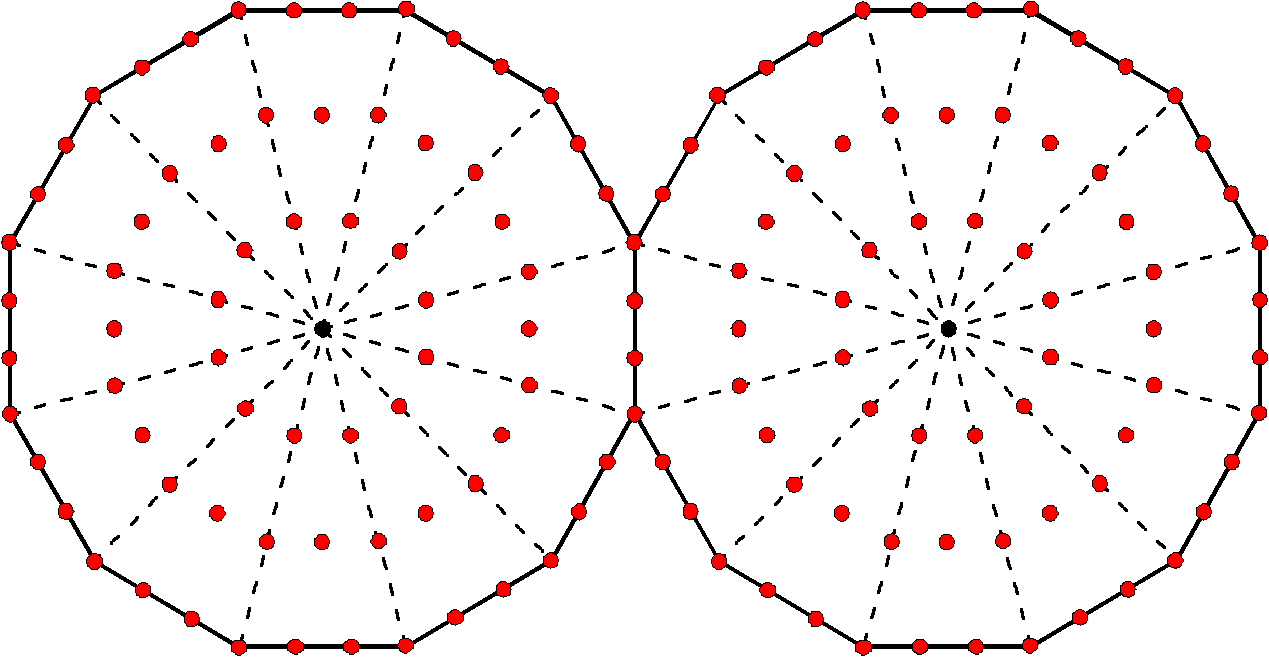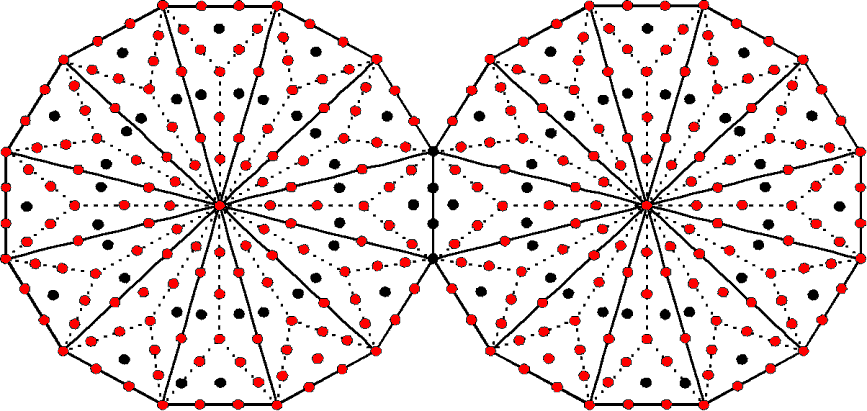The Type A dodecagon contains 73 yods. This is the number of Chokmah, meaning "wisdom." 72 yods surround the centre of the Type A dodecagon. This is the number of Chesed. 140  yods surround the centres of 2 Type A dodecagons. This is the number of Masloth , the Mundane Chakra of Chokmah. Its astrological counterpart in Kabbalah is the Zodiac. 282 yods line the 72 tetractyses in 2 Type B dodecagons. This is the number of Aralim, the Order of Angels of Binah.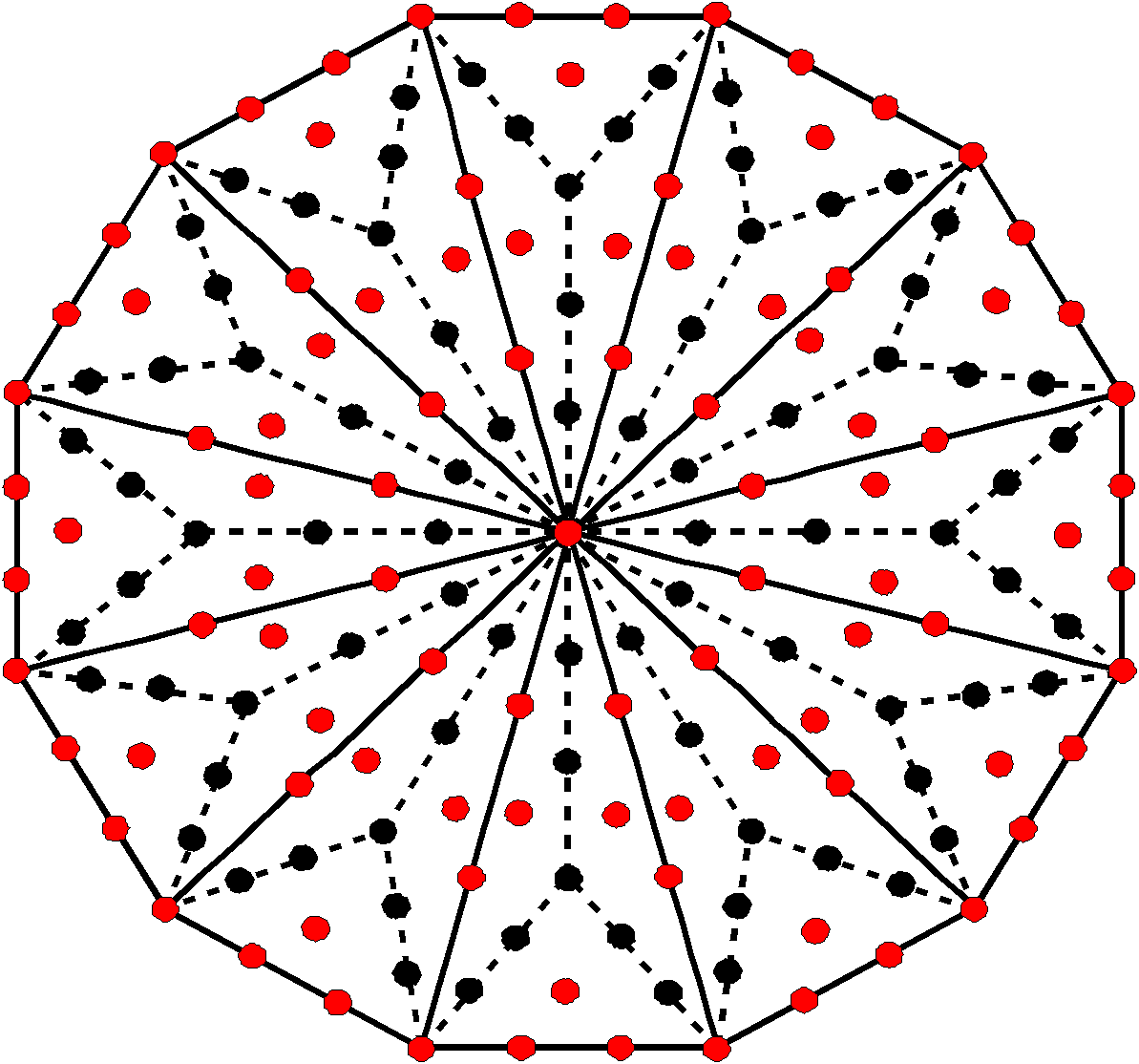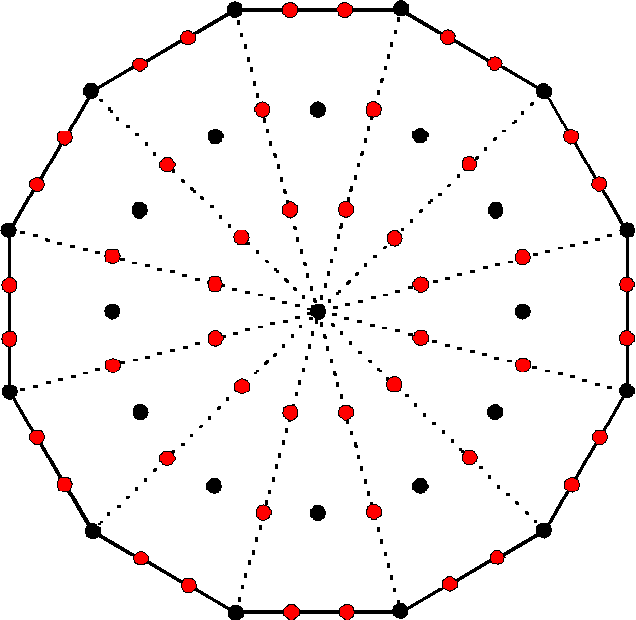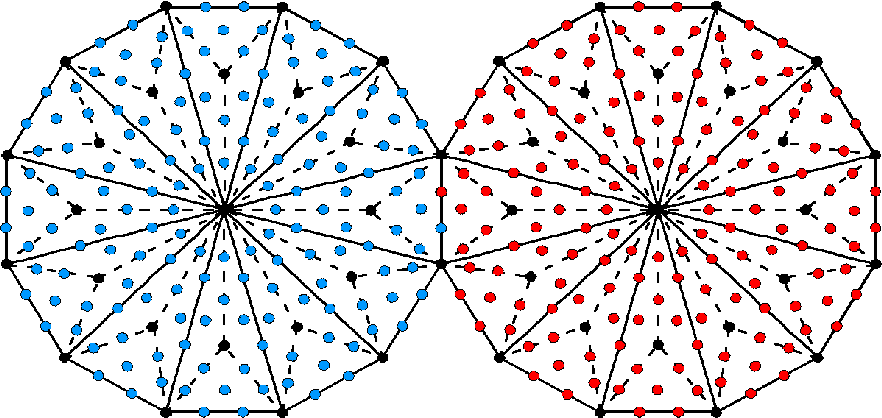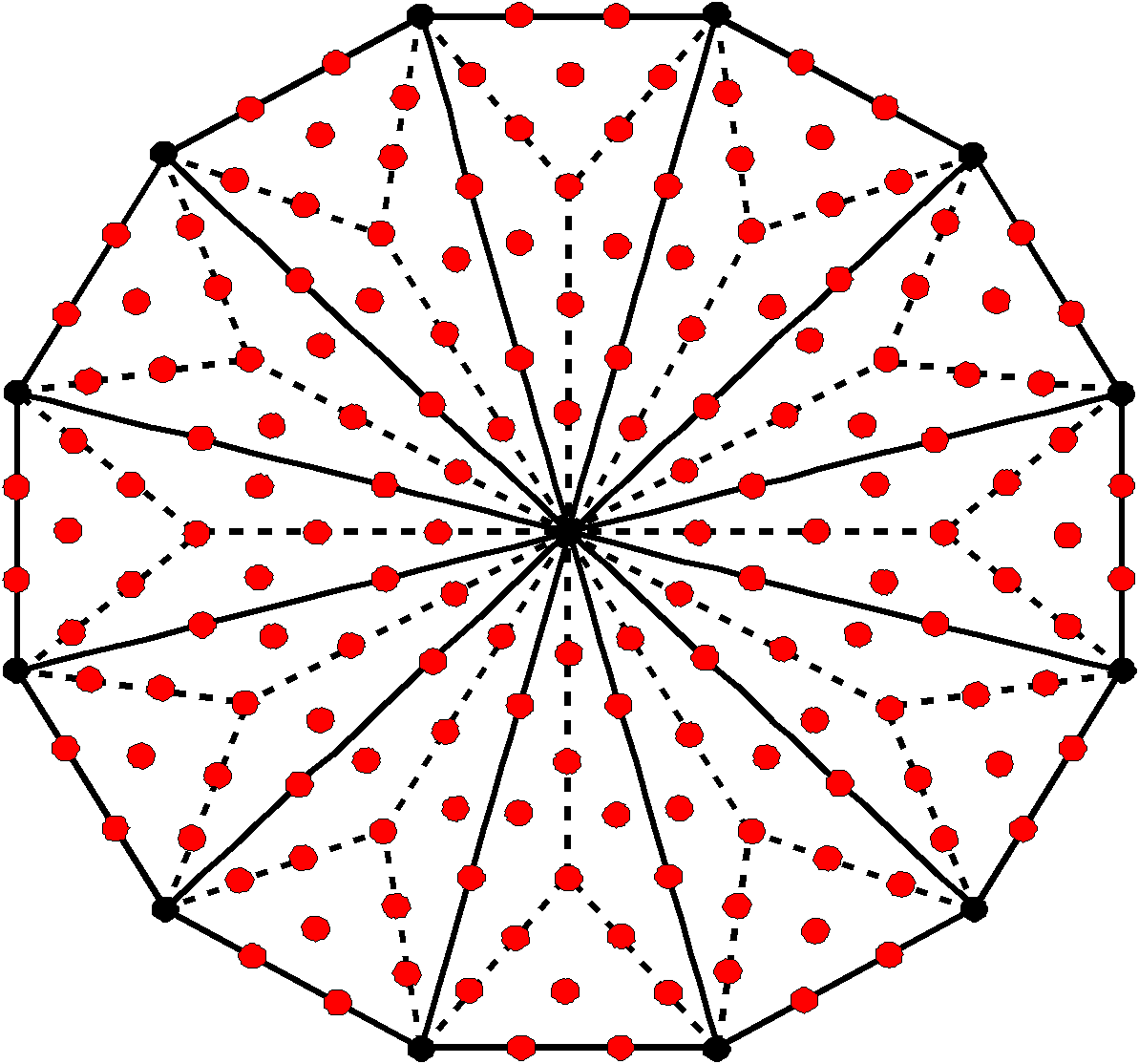97 yods either line sectors or are at centres of tetractyses in the Type B dodecagon. This is the number of Haniel, the Archangel of Netzach. 48 hexagonal yods line the sides of the sectors of a Type A dodecagon. This is the number of Kokab, the Mundane Chakra of Hod. 155 red or blue hexagonal yods are associated with each Type B dodecagon. This is the number of ADONAI MELEKH, the Godname of Malkuth. 168 yods other than its corners surround the centre of the Type B dodecagon. This is the number value of Cholem Yesodeth, which is the Mundane Chakra of Malkuth. Figure 8. The wisdom of the dodecagon.

8

reason why this happens is that the tetractys-divided Type A dodecagon is a representation of 12 overlapping Trees of Life, its 73 yods corresponding to the 73 SLs up to Chesed of the 12th Tree. When it is Type B, the 180 yods surrounding its centre symbolise the 180 years that elapse during 1/12th of the precession of the equinox through the 30 degree arc of an astrological sign of the 12-fold Zodiac, which is symbolised by the dodecagon. The 2160 such yods surrounding the centres of the Type B dodecagons enfolded in 12 overlapping Trees symbolise the 2160 years required for the Earth's axis to rotate through 30° to complete the journey through an astrological sign. The complete precessional cycle is mapped by 144 Trees — the counterpart of the 144 sectors of the dodecagons enfolded in 12 Trees. This number was encountered in the discussion in the Polyhedral Tree of Life subsection of Sacred geometry as the number of yods that line the sides of the seven separate regular polygons making up each half of the inner Tree of Life (see here). When they become enfolded, the polygons are lined by 120 yods but there are 144 yods inside their boundaries. This 120:144 distinction between the exterior and interior of the inner Tree of Life manifests in 3-dimensional space as the 120 faces of the disdyakis triacontahedron and the 144 faces of what has been called the "144 Polyhedron," both polyhedra discussed in that subsection as, respectively, the outer and inner forms of the Polyhedral Tree of Life. Remarkably, there are 144 SLs on the Pillar of Equilibrium of CTOL below the bottom of the 73rd Tree! Equally remarkable is the fact that 144 yods inside the Type B dodecagon surround its centre. This, of course, is no coincidence, for the 73 yods in the Type A dodecagon symbolise SLs, which can be represented by complete Trees of Life. One may say, therefore, that the 72 yods surrounding its centre symbolise the 72 Trees below the 73rd Tree that have (72×2=144) SLs on the central pillar below its Tiphareth symbolised by the 144 internal yods, there being two such SLs per Tree. The 144th SL on the Pillar of Judgement is Geburah of the 72nd Tree. It is the 432nd SL and emanates 145 SLs on the central pillar and 143 SLs on the Pillar of Mercy.

What is significant is not so much the ancient division of the celestial sphere into 12 segments containing constellations but that the division of the precessional cycle into n equal stages conforms to a holistic pattern only when n = 12. As the number of yods in an n-sided, regular polygon = 15n + 1, the number of yods surrounding the centres of the n n-sided polygons enfolded in the n-tree = n×15n = 15n2. This equals 2160 only when n = 12. The dodecagon is unique in being the only polygon that embodies this number when the number of copies of the polygon is identical to the number of its sectors. The first four enfolded polygons have the same yod population (making allowance for their shared corners) only when both sets are considered. If the centre were included, then n×(15n+1) = 2160. But this quadratic equation has no integer solution for n, so only the yods surrounding the centre of the dodecagon provide a valid, unique representation of the number 2160. But, as the polygonal representation of the Tree of Life, it embodies other Kabbalistic numbers. Figure 8 shows how the dodecagon embodies some of the gematria numbers of the 10 Sephiroth in the four Kabbalistic worlds, as listed in Table 1. Notice in particular that 140 yods surround the centres of two joined, Type A dodecagons, where 140 is the number value of Masloth (3), the Mundane Chakra of Chokmah, the Sephirah whose number 73 is the yod population of a single dodecagon. This is appropriate, given that it embodies the astrological age of 2160 years, because Kabbalists assign the Sphere of the Zodiac to Masloth!

Is there any astronomical significance to a rotation of Earth's axis by 2½° over a period of 180 years that corresponds to a complete Tree of Life, the Platonic Year corresponding to 144 overlapping Trees of Life? The 180 yods in a Type B dodecagon that surround its centre consist of the 72 yods in a Type A dodecagon and the 108 yods added by transforming it into the next type. As a yod symbolises a year, the former number, which is 2/5 of 180, signify a rotation of (2/5)×(5/2) = 1° over 72 years, whilst the latter encodes a further rotation of 1½° over 108 years. Is there any astronomical evidence for the 30° rotation of Earth's axis during an astrological age of 2160 years dividing into a rotation of 12° over 864 years and a rotation of 18° over 1296 years (or 144° (10,368 years) & 216° (15,552 years) for the Platonic Year of 25,920 years? In other words, for the 360° rotation being a five-fold cycle that is divided in the proportion of 2:3?

Before answering these questions, the issue of numerology must be addressed. Firstly, the Moon's diameter is often quoted as 2160 miles, although this is an approximation because it is not perfectly spherical, the more accurate mean diameter being 2159.2 miles. The precession of Earth's axis is well understood as due to the combined gravitational, tidal force of the Sun and Moon acting on Earth's equatorial bulge, although unequally on the part above and below the plane of its orbit to which it is tilted (a difference that generates a torque continually twisting its axis in a circle). However, it remains an amazing 'coincidence' that the length in years of an astrological age that this torque causes and the diameter of the Moon measured in miles should be almost exactly identical numbers! Of course, it has to be no more than that, a scientist will argue, because there can be no causal connection between the rate of wobble of the Earth and the size of a piece of rock either captured in the past by the Earth or ejected from it whilst it was in a molten state. He will point out that the force of gravity between the Moon and Earth that is partly responsible for the precession depends only on the mass and distance of the former,

9

not on its size, so the periodicity of the rotation of Earth's axis cannot be related to the Moon's diameter. However, although perfectly true, this may not be the point. If universal laws of nature applied to analogous systems produce features patterned by the same set of numbers, why should we always need to demand a physical explanation, the absence of which necessarily means that the appearance of the number 2160 in different astronomical contexts must be merely a coincidence? Perhaps nature works through subtle, as yet undiscovered, mathematical laws of analogy or correspondence between systems, whatever the difference in scale between them — laws that are even more fundamental than Newton's laws of motion and gravity, but acting acausally instead of mechanistically. Secondly, the actual period of the precession of the equinox is about 25,772 years, not 25,920 years. This amounts to one degree of rotation of Earth's axis every 71.6 years, not exactly 72 years, and to an astrological age of 2147.7 years, not 2160 years. Do these slight numerical differences invalidate comparison with sacred geometry? Not at all, for many small, astronomical perturbations are at work, making the actual figures deviate from the theoretical ideal. For example, the gravitational pull of planets, particularly Jupiter, creates a small precessional motion that is added to that caused by the Sun and Moon. Moreover, the actual rate of precession is not constant but (at the moment) slowly increases over time. This, too, has to be taken into account when comparing the differences between theoretical values based upon sacred geometry and scientifically measured values. It is a clouded situation that will be familiar to any scientist who has compared his experimental data with the predictions of some theory that he is testing. If their discrepancies are too large on average, statistically speaking, to be due to errors of measurement, he will conclude that his data does not support the theory — but only if he has satisfied himself that he eliminated or minimised all laboratory variables that might disturb his measurement. What has to be assessed is whether various theoretical and measured values agree too closely and too often to be plausibly due to chance. A discrepancy in the rate of rotation of Earth's axis as small as 0.5% is hardly to be ignored. Despite this degree of agreement, scientists will dismiss it because too many of them are locked into an ideology that rejects regularities — even phenomena — as real if science cannot provide a physical explanation for them. For example, this has been (and continues to be for many astronomers) the fate of the Titius-Bode law (see Article 17). Because they reject acausal explanations as unscientific, they remain unconvinced by the beautiful properties of sacred geometries, however amazingly these embody astronomical parameters. Their narrow philosophy of scientism will not permit them to accept the possibility that cosmic and small-scale phenomena might exhibit the same, mathematical patterns because they are governed by the same, non-mechanistic principles, being realizations of the same cosmic Whole. For most scientists, there is no overriding Whole, only unconnected fragments randomly scattered about in a universe of chance events. Hence, they dismiss any evidence contradicting this as the product of coincidence.

Let us return to the issue of whether a five-fold structure can be discerned in either the Platonic Year or an astrological age. The number 5 suggests the relevance of the ancient teaching of the five elements. Western astrology, however, relates the 12 astrological signs to the four physical elements of ancient Greek philosophy, there being three signs associated with each element and no sign corresponding to the fifth element Aether (Table 3):

Table 3. Assignment of the four physical elements to the 12 astrological signs.

 Aries Fire Taurus Earth Gemini Air Cancer Water Leo Fire Virgo Earth Libra Air Scorpio Water Sagittarius Fire Caprican Earth Aquarius Air Pisces Water

The Chinese yearly calendar does, however, have a 5-fold character because it has five "seasons" or divisions of the year (four traditional seasons and an intermediate, representing the change between two successive seasons). In their correct order, the five Chinese elements (Wu Xing) are: Wood, Fire, Earth, Metal & Water. Rather than substances or states of matter, these words should be understood as metaphors for the five stages or phases of a cyclical process (in this context the passage of a year). The words "Fire", Earth" & "Water" must not be taken literally, nor must they be confused with the ancient names of the physical elements. They are called 'elements' only through the influence of the western

10

philosophy of the elements. The association of Wood with Spring expresses the growth (sprouting) stage of a life process, Fire associated with Summer represents the flowering or blooming phase, Earth's association with Late Summer denotes the fruition, or ripening, stage, Metal's association with Autumn denotes the harvesting, collecting, withering stage and Water associated with Winter expresses the retreat, stillness or dormancy of life. There are six Yang and six Yin Earthly Branches.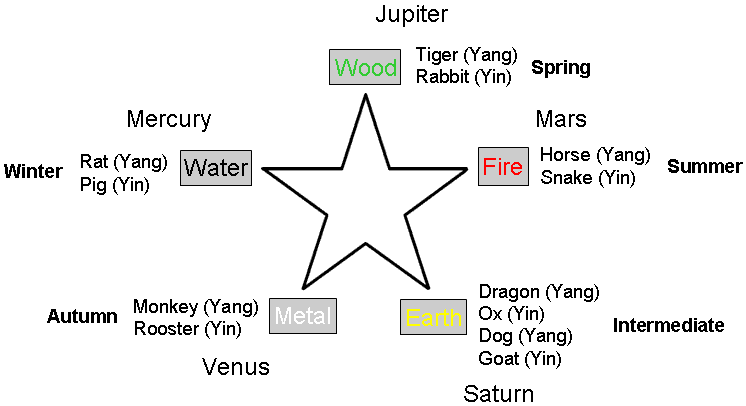Figure 9. Correspondence of elements and seasons to the 12 Earthly Branches.

The 5-fold division of the 12 Earthly Branches is achieved by assigning a pair of branches (Yang & Yin) to each of the four elements Wood, Fire, Metal & Water and two pairs to the fifth element Earth (Fig. 9). A Yang branch is always followed by a Yin branch and vice versa (Table 4):

Table 4. The 12 Earthly Branches & their elements.

 12 Earthly Branches Element 1 Rat Yang Water 2 Ox Yin Earth 3 Tiger Yang Wood 4 Rabbit Yin Wood 5 Dragon Yang Earth 6 Snake Yin Fire 7 Horse Yang Fire 8 Goat Yin Earth 9 Monkey Yang Metal 10 Rooster Yin Metal 11 Dog Yang Earth 12 Pig Yin Water

The years are grouped within a 10-year cycle called the "10 Celestial Stems". Each year corresponds to one of the five elements (Yang or Yin forms). There is also a 12-year cycle called the "12 Earthly Branches" based upon 12 animal signs and a 60-year cycle called the "stem-branches" in which the Celestial Stems and the Earthly Branches cycle run together so that a given animal sign repeats itself five times over 60 years, once for each element every 12 years (for comparison with the western calendar, see here). We see here parallels with how the holistic parameter 2160 is embodied in different ways in both 10 and 12 overlapping Trees of Life, as described above. Although the Chinese have a five-fold division in a year, it refers, not to equal periods of time, but to types of temporal change in the life process, as manifested within the four traditional seasons and in the transition from one to another. It is, therefore, misconceived to look for some even, five-fold astronomical pattern within a year. It does, however, exist in an astrological era and the Platonic Year because both are exactly divisible by 60. The former is composed of (36×5=180) repetitions of each zodiac animal sign and the latter comprises (12×180=2160) repetitions. We see that the ancient Chinese calendar contains the same number 2160 when extended over a complete processional cycle! However, it refers not to a period of time but to the number of times each animal sign is repeated in this cycle.

The 180 yods surrounding the centre of a Type B dodecagon symbolise the 180 years required for the Earth's axis to rotate through 2½°, or 1/144th of a circle. The 72 yods surrounding the centre of a Type A dodecagon denote the 72 years that elapse during its rotation by 1°, or 1/360th of a circle. The extra 108 yods in a Type B dodecagon represent the 108 years during which the axis rotates by 1½°, or 1/240th of a circle. In one year, the axis should rotate through 1/72° = 50″, an angle that corresponds to a yod, whilst

11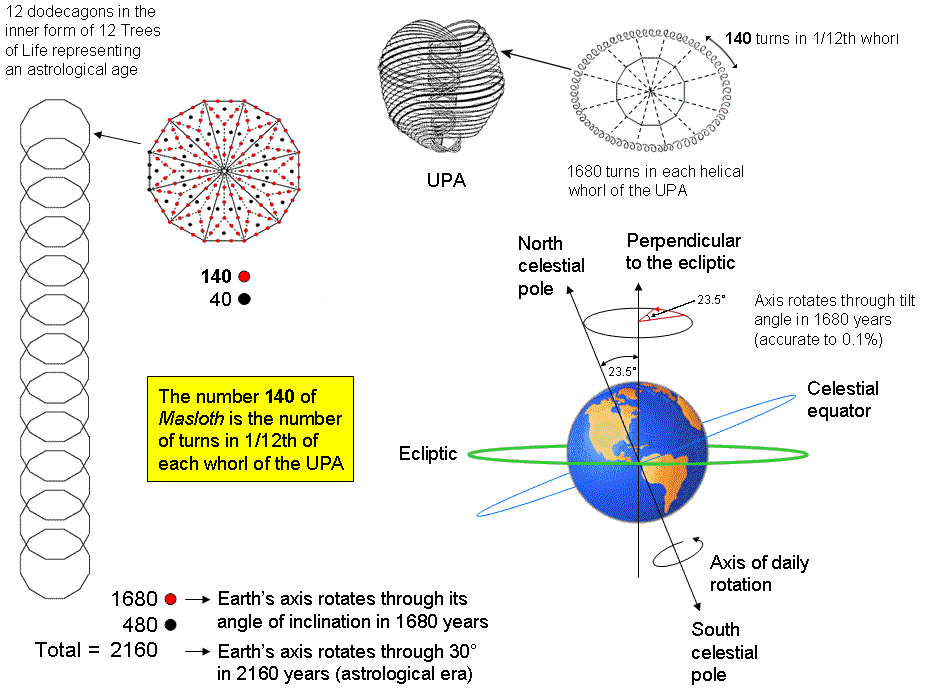Figure 10. Is it just coincidence that the gematria number 140 of Masloth, the Mundane Chakra of Chokmah and the Kabbalistic name for the 12-fold Zodiac, is the number of turns in 1/12th of a whorl of the UPA/superstring, whilst 168, the number of the Mundane Chakra of Malkuth (physical universe) is what determines the number (1680) of turns in the whole whorl?

12

it should rotate through 50° in 3600 (=36×10×10) years. The number value 50 of ELOHIM, the Godname of Binah, is the number of arcseconds through which the Earth's axis should rotate in one year (the actual value is 50.3", a discrepancy of about 0.6% that is small enough to regard this prediction as significant). The number value 36 of ELOHA, the Godname of Geburah, which is the Sephirah directly below Binah in the Tree of Life, determines the time taken for it to rotate 50°. The appearance here of the numbers 50 and 36, as well as the numbers 72 and 216 elsewhere, where 72 is the number of Chesed and 216 is the gematria number of Geburah, is difficult to dismiss as coincidence, given that Binah, Chesed & Geburah are successive Sephiroth in the Tree of Life.

Finally, the following amusing curiosity is offered: how much does the earth's axis rotate during 1680 years, where 1680 is the number of circularly polarised oscillations in each helical whorl of the UPA, as counted by C.W. Leadbeater over a century ago when he used a form of remote viewing to describe subatomic particles? This website proves beyond all reasonable doubt that this number is a parameter so conspicuously and naturally embodied in sacred geometries that it renders untenable the kind of vacuous criticism of cherry-picking that sceptics might resort to in order to dismiss Leadbeater's work. So it is interesting to know what angle the Earth's axis would rotate through in 1680 years about a line perpendicular to the ecliptic. Assuming the actual value of the period of the precession of the equinox, i.e., 25,772 years, as opposed to its idealised value of 25,920 years, the axis of the Earth rotates through an angle of about 23.46733° in 1680 years. Compare this with its angle of tilt of about 23.43928° from the perpendicular to the plane of the Ecliptic. Earth's axial tilt varies from 22.1° to 24.5° over a 42,000 year period. The tilt is currently decreasing. The difference between the current angle of tilt and the angle the axis would rotate through in 1680 years if its rate of rotation remained constant is about 0.12%. This should be small enough to induce at least an embarrassed smile in the sceptic before he hastily dismisses it as yet another coincidence! The significance of the superstring structural parameter 1680 in the context of the Type B dodecagon with 180 yods in 36 tetractyses surrounding its centre is that (180−36=144) of these yods line their 60 sides, i.e., (144−4=140) boundary yods are outside the root edge, where 140 is the gematria number value of Masloth (The Sphere of the Zodiac), which is the Mundane Chakra of Chokmah (see Table 1). (12×140=1680) yods delineate the (12×36=432) tetractyses making up the 12 Type B dodecagons enfolded in 12 Trees, whereas the remaining 480 yods either belong to their root edges or are hexagonal yods at the centres of these tetractyses. This demonstrates the shape-forming character of the number 1680, as realised in the 1680 circular turns of each helical whorl of the UPA/subquark superstring (see here). The number of Masloth is the number of circular turns in 1/12th of a whorl of the UPA (Fig. 10). The 12-fold aspect of the Zodiac has its counterpart in each whorl of the subquark superstring, just as the five Chinese elements, or stages of a life cycle, correspond to the five revolutions or twists in each whorl about the axis of spin of the UPA. This accords with the Hermetic axiom: "As above, so below."

References

1. A perfect number is an integer that is the sum of all its divisors. For example, the number 6 is the first perfect number because 6 = 1 + 2 + 3, where 1, 2 & 3 are its divisors, and 28 is the second perfect number because 28 = 1 + 2 + 4 + 7 + 14, where 1, 2, 4, 7 & 14 are its divisors.

2. The zodiacal signs are the twelve 30° sectors of the ecliptic and need to be distinguished from the 12 constellations of the zodiac, which take up varying amount of ecliptic longitude, e.g., the constellation of Virgo takes up five time as much longitude as the constellation of Scorpius. This means that the Sun is not in each constellation for the same amount of time.

3. Masloth is also written as Mazloth, with the Hebrew letter zayin being used instead of samekh. Its gematria value is then 483. The present geometrical context makes it clear that 'Masloth' is the right spelling, with the number 400 contracted to 4: MSLVTh = 40 + 60 + 30 + 6 + 400→4 = 140.

13

Home